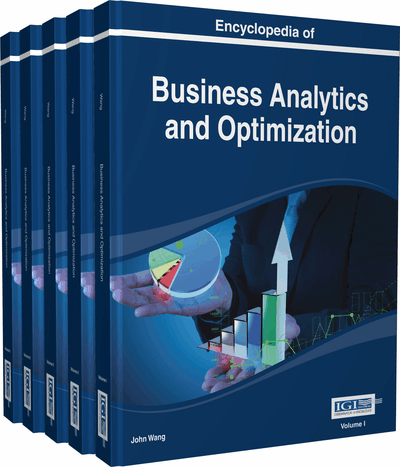# Multiobjective Programming

Minghe Sun (College of Business, University of Texas at San Antonio, USA)
DOI: 10.4018/978-1-4666-5202-6.ch143

## Abstract

Optimization problems with multiple criteria measuring solution quality can be modeled as multiobjective programming problems. Because the objective functions are usually in conflict, there is not a single feasible solution that can optimize all objective functions simultaneously. An optimal solution is one that is most preferred by the decision maker (DM) among all feasible solutions. An optimal solution must be nondominated but a multiobjective programming problem may have, possibly infinitely, many nondominated solutions. Therefore, tradeoffs must be made in searching for an optimal solution. Hence, the DM's preference information is elicited and used when a multiobjective programming problem is solved. The model, concepts and definitions of multiobjective programming are presented and solution methods are briefly discussed. Examples are used to demonstrate the concepts and solution methods. Graphics are used in these examples to facilitate understanding.

## Key Terms in this Chapter

Interactive Procedure: A solution procedure in which there are two alternate phases, a solution generation phase and a solution evaluation phase.

Pareto Optimal Solution: A nondominated solution in the criterion space or an efficient solution in the decision space.

Optimal Solution: A solution that maximize the DM’s value function or that is most preferred by the DM.

Nondominated Solution: A solution in terms of the values of the objective functions where any objective function cannot be improved without deteriorating one or more other objective functions.

Feasible Region in Decision Space: The set of all solutions in terms of the values of the decision variables satisfying all constraints.

Efficient Solution: A solution in terms of the values of the decision variables where any objective function cannot be improved without deteriorating one or more other objective functions.

Feasible Region in Criterion Space: The set of all solutions in terms of the values of the objective functions satisfying all constraints.

Multiobjective Programming Model: a mathematical programming model with more than one objective function.

## Complete Chapter List

Search this Book:
Reset